# Block Placed on Top of Another Block - Finding Max Force

• The Physics Moss

## Homework Statement

[/B]
A 0.5kg wooden block is placed on top of a 1.0kg wooden block. The coefficient of static friction between the two blocks is 0.35. The coefficient of kinetic friction between the lower block and the level table is 0.20.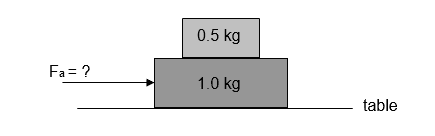What is the maximum horizontal force that can be applied to the lower block without the upper block slipping?

Fnet = ma
Fk = μkmg
Fs = μsmg

## The Attempt at a Solution

I understand that the acceleration of both blocks will have to be the same for the top block to not slip. However, I have problems understanding the free-body diagrams given in the final solution compared to the ones in my attempt at the solution.

These are the free body diagrams given in the final solution:
Top Block: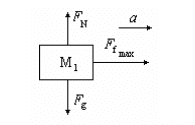Whole System: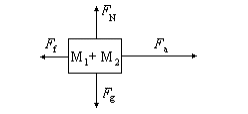By Ff max they mean static friction and by Ff they mean kinetic friction

What I do not understand is:
1) Why is the applied force in the system diagram if it is only being applied to the bottom block?
2) Why does the system diagram include only kinetic friction when it is only being applied to the bottom block?
3) Why are the forces the boxes are exerting on one another not included?

In my attempt at solving this question, I drew two free-body diagrams:
Top Block: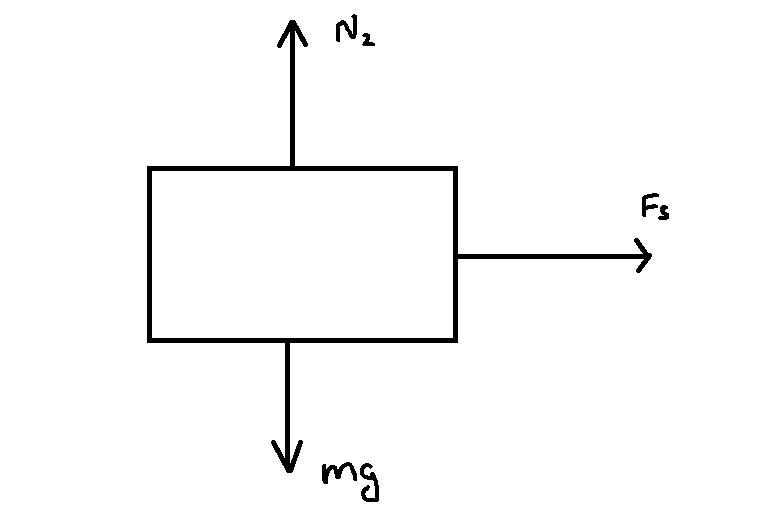Bottom Block: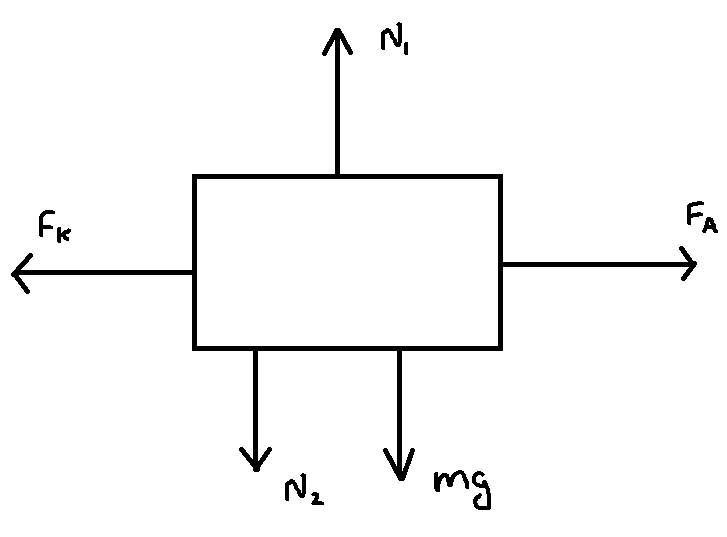As mentioned before, my attempt gave me the wrong answer. Also, through some research, I found solutions to similar questions with free-body diagrams showing the static friction between the two blocks also affecting the bottom block in addition to the kinetic friction (both in the left direction). I'm not sure how that would work.

Last edited:
What I do not understand is:
1) Why is the applied force in the system diagram if it is only being applied to the bottom block?
2) Why does the system diagram include only kinetic friction when it is only being applied to the bottom block?
3) Why are the forces the boxes are exerting on one another not included?
i think one must imagine that the system of two blocks is moving with acceleration say a by an external force being applied.

1.as the bottom block is moving along with the top block and one has to find the surface on which it would move.(if the force is applied on top one the situation will be different,which is not being asked to analyze) condition for the top one not to slip with respect to bottom so it would be proper to move it by pushing the bottom one in contact with the

2. when the two block system is moving the surfaces in contact and in relative motion is the bottom surface , therefore the kinetic friction acts opposite to the motion .therefore this is logical -no other forces are there to oppose the motion of the system.

3.The forces in between the surfaces of the two blocks are internal forces and by Newton's third law the force on bottom by the top block and the force on the top by the bottom block along the surface of contact due to static friction must be equal and opposite.

•The Physics Moss
s mentioned before, my attempt gave me the wrong answer. Also, through some research, I found solutions to similar questions with free-body diagrams showing the static friction between the two blocks also affecting the bottom block in addition to the kinetic friction (both in the left direction). I'm not sure how that would work.

i think your free body diagram needs correction;
if the system is moving to the right by say acceleration a , then the top block will experience a frictional force in the forward direction which will oppose the top block being left behind and if it can not counter it the top one will slip behind - so there is a limit to the acceleration of the system.
the frictional force is always opposed to the tendency of motion of the surfaces in contact.
if you take the system as a whole the kinetic friction will be acting opposite to the motion of the blocks at the surface in contact

if you consider bottom block separately then its weight will become total weight of the system and the kinetic friction force will oppose it.
the reaction of the bottom on the top block will be equal to the wt of the top on the bottom.therefore the bottom will be heavier by addition of the top's weight and total reaction felt by the bottom will be equal to the total weight.mind it action and reaction forces are equal and opposite but act on different bodies .

i think your free body diagram needs correction;
if the system is moving to the right by say acceleration a , then the top block will experience a frictional force in the forward direction which will oppose the top block being left behind and if it can not counter it the top one will slip behind
That's what the FBD for the top block shows. But there should be a corresponding Fs to the left on the lower block, as well as the Fk from the ground.

That's what the FBD for the top block shows. But there should be a corresponding Fs to the left on the lower block, as well as the Fk from the ground.

Can you please explain why this is the case?

Can you please explain why this is the case?
Newton's law of action and reaction. If the lower block is exerting a static friction force to the right on the top block then the top block must be exerting an equal and opposite force on the lower block.